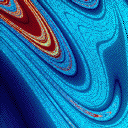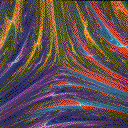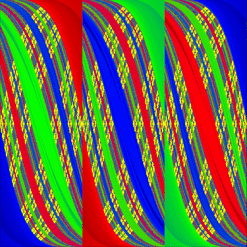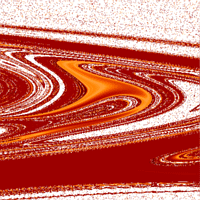# Basin Pictures

## Forced Damped Pendulum

...with Four Basins
Even something as simple as a periodically forced damped pendulum can have complex behavior. The computer-generated images below show initial positions that asymptote to one of different behaviors (one color for each behavior). For example, orbits starting at points in the blue region would yield a different type of motion from orbits starting in the red region. The brighter the shade of color, the longer it takes to settle into the corresponding motion. The different regions are separated by fractal basin boundaries. The pictures are made at increasing magnification levels.

...with Two Basins
Same as above except the parameter values are slightly different so there is only two attractors in this case instead of four. Here, lighter shades of color represent points that settle down more slowly.

```(109K)(150K)
```

## Fractal Foam

There are three simple attractors (straight lines in fact!) which formed sort of a triangle; however, the basins of attraction is anything but simple. These pictures are the starting points for the interesting ideas of riddled basins.
```(82K)(66K)
```

```(373K)
```(78K)